Courses
Courses for Kids
Free study material
Free LIVE classes
More

# 2 Digit Subtraction on Number LineLIVE
Join Vedantu’s FREE Mastercalss

## Overview of Subtraction on Number Line

Mathematically, subtraction is considered one of the four arithmetic operations. In our day-to-day life, we can observe and see the applications of subtractions in different situations. For example, every day, we purchase vegetables and fruits for a certain amount, say Rs. 175, and we have given a note of Rs. 200 to the vendor.

So, now the vendor has to return the excess amount by applying the process of subtraction which will be 200 - 175 = 25. Therefore, the vendor is obligated to return Rs 25. Similarly, number line subtraction worksheets help us to perform the subtraction operation on small numbers visually.

## What is Subtraction on the Number Line?

Subtraction on the number line helps to subtract the smaller numbers in an easy manner or way. While subtracting numbers on a number line, it is necessary or compulsory to jump forward towards the left-hand side of a number line. Under this, we always begin with the minuend and then we move left towards the number accordingly given as the subtrahend.

• Minuend: The number from which the other number is subtracted.

• Subtrahend: The number which is to be subtracted from the minuend.

• Difference: The final result after subtracting the subtrahend from the minuend.

For example:

Here we are subtracting 2 from 4 using a number line.

According to the formula, Difference = Minuend - Subtrahend.

Thus, 4 - 2 = 2.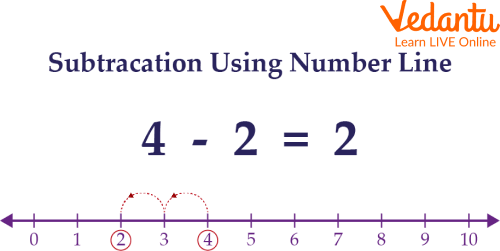Subtraction using the number line

Let us take a detailed stepwise process of subtraction using the number line.

Step 1: Firstly, mark the Minuend (4) on the number line.

Step 2: Move forward towards the left-hand side of the number according to the number provided in the subtrahend. In this scenario, the subtrahend is 2, so we will move 2 units forward to the left. Hence, we land on 2, which is the answer to our problem.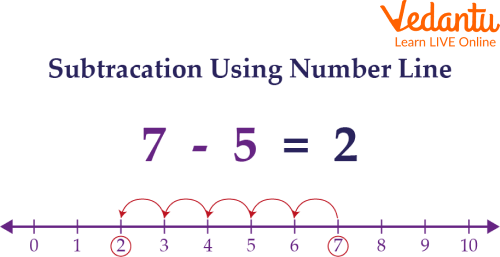Another example of subtraction using the number line.

## 2-Digit Subtraction on a Number Line

Now, we are going to understand 2-digit subtraction by using an open number line which is followed by a certain set of rules. An open number line can be defined as an empty number line with no number marked on it.

For example: Subtract 45 from 78 using a number line.

As we know, 78 - 45 = 33. Now, we are going to solve this by using a number line.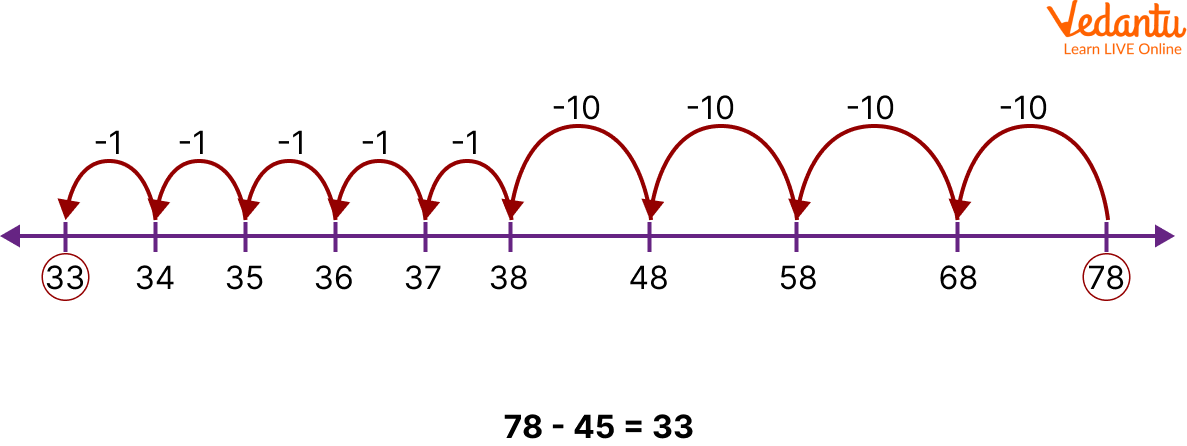Subtraction using the number line

Step 1: First, mark the minuend (78) on the open number as shown in the figure mentioned above.

Step 2: Now, count the number of tens and ones in the subtrahend (45), after counting we have 4 tens and 5 ones.

Step 3: Then, move forward towards the left according to the number of tens (taking longer jumps) which is obtained in the previous step and mark the numbers simultaneously after every move (68, 58, 48,........33) on the number line. The last number we arrive at will be the difference (33).

Lastly, we get the result,

78 - 45 = 33 by using a number line for 2-digit subtraction.

## Solved Examples

Below given are some solved examples based on the number line subtraction worksheets:

Q1. Perform the subtraction on the number line for the given expression: 19 - 15 = 4.

Ans: Let us determine the difference between 9 and 5 by subtracting the number line.

We start by marking the number 19 on the number line. Then we move forward towards the left by 4 units, and we reach 15.

Therefore, we get the result 19 - 15 = 4.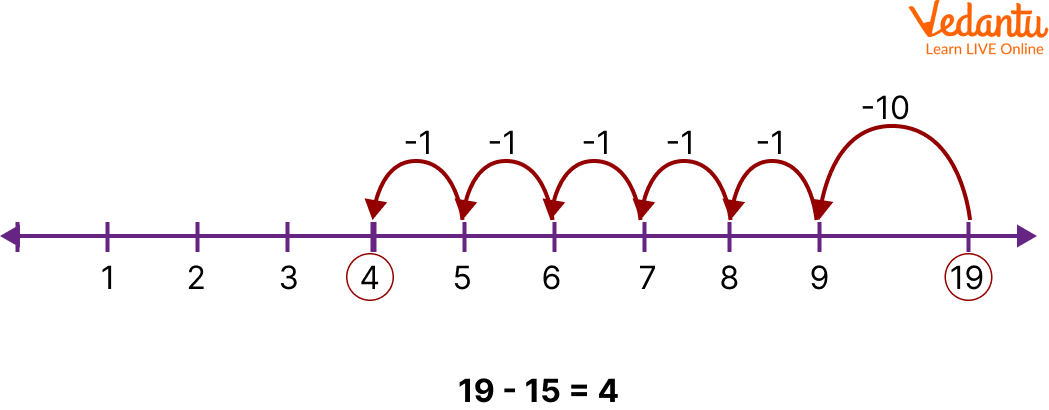Subtraction using the number line

Q2. Subtract 29 from 54 using 2-digit subtraction on a number line.

Ans: Let us draw an open number line as shown below and follow the steps of 2-digit subtraction on the number line.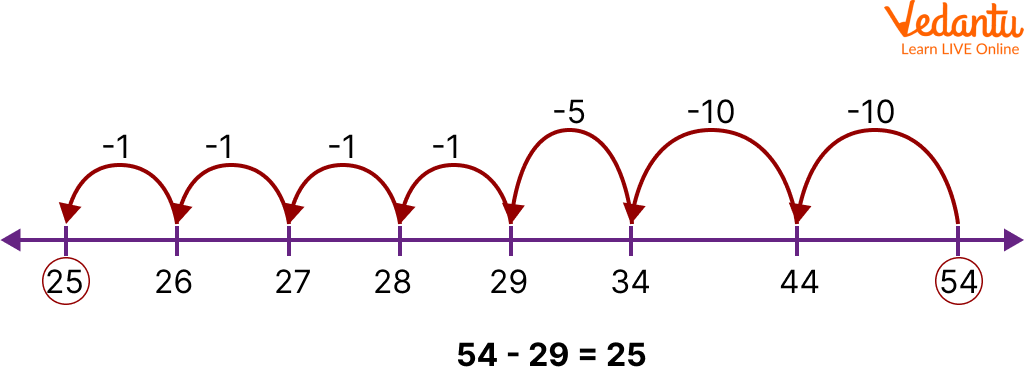Subtraction using the number line

1. Consider the first number 54 and then mark it on the open number line.

2. Here, the second number is 29, which means it has 2 tens and 9 ones.

3. Then take 54 as the starting point, and we will be moving 2 tens (longer jumps) and 9 ones (shorter jumps) towards the left, which is shown in the figure.

Therefore, we get the result of 25 on solving 54 - 29.

Q3. What is the difference between the largest 2-digit number and the number 20?

Ans: The largest 2-digit number is 99.

The given number is 20.

So, the difference is given by,

99 - 20 = 79.

Thus, the difference between the two numbers is 79.

## Practice Questions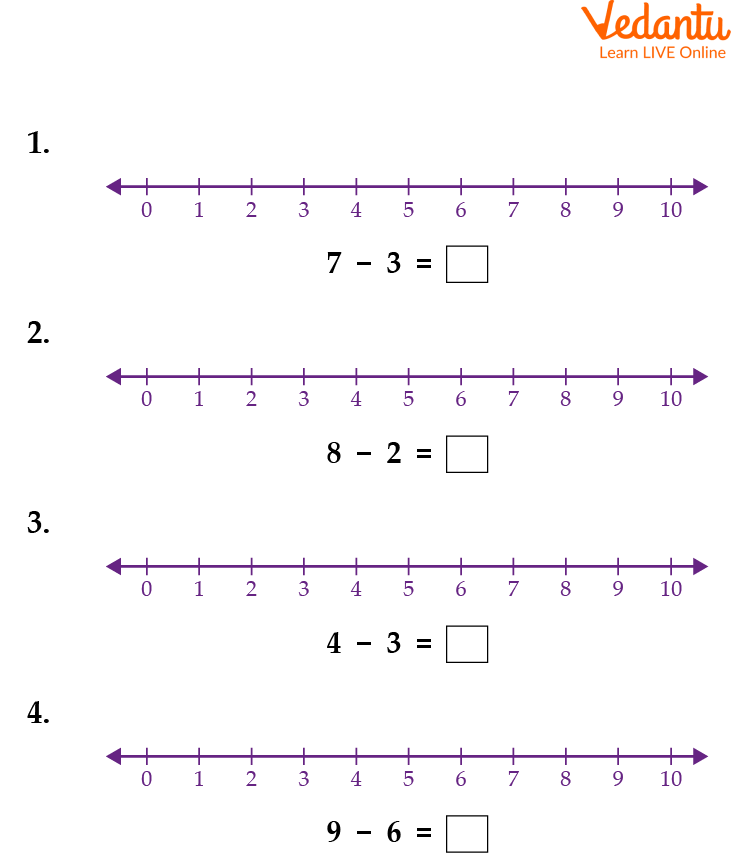Number line subtraction worksheets

1. 4

2. 6

3. 1

4. 3

## Summary

In this chapter, we have dealt with subtraction on the number line and the 2-digit subtraction number line worksheet. A number line is the pictorial representation of numbers on a straight line. The numbers on the number line increase as one move from left to right and decrease on moving from right to left. A number line can perform different arithmetic operations such as addition, subtraction, multiplication and division. The 2-digit subtraction number line worksheet helps evaluate the children's understanding.

Last updated date: 28th Sep 2023
Total views: 70.5k
Views today: 0.70k

## FAQs on 2 Digit Subtraction on Number Line

1. How many numbers can be represented in a number line?

Infinitely, many numbers can be represented on a number line as it can be extended on both sides.

2. How to subtract negative numbers on a number line?

When negative numbers are subtracted by using a number line, then we move towards the right-hand side because, according to the rules of integers, when a negative number is subtracted, the operation turns into addition.

3. How are the negative and the positive integers placed on a number line?

The positive integers are placed to the right of 0, and the negative integers are placed to the left of 0.